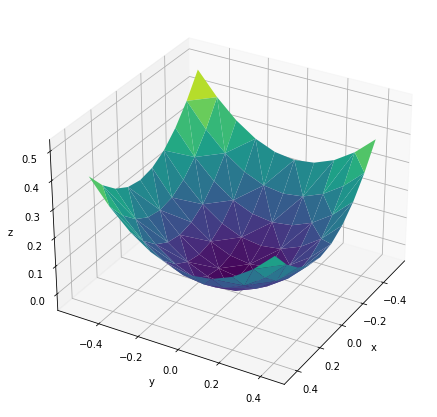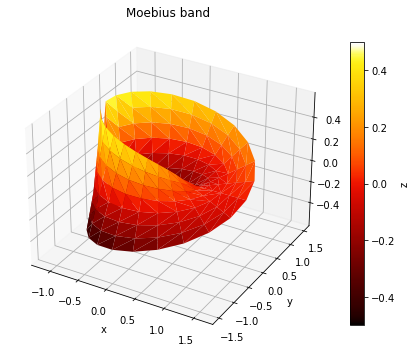# TriSurface#

Title
TriSurface Element
Dependencies
Matplotlib
Backends
Matplotlib
Plotly
```import numpy as np
import holoviews as hv
hv.extension('matplotlib')
```The `TriSurface` Element renders any collection of 3D points as a surface by applying Delaunay triangulation. It is therefore useful for plotting an arbitrary collection of datapoints as a 3D surface. Like other 3D elements it supports `azimuth`, `elevation` and `distance` plot options to control the camera position:

```y,x = np.mgrid[-5:5, -5:5] * 0.1
heights = np.sin(x**2+y**2)
hv.TriSurface((x.flat,y.flat,heights.flat)).opts(
azimuth=30, elevation=30, fig_size=200)
```Like all other colormapped plots we can easily add a `colorbar` and control the `cmap` of the plot:

```u=np.linspace(0,2*np.pi, 24)
v=np.linspace(-1,1, 8)
u,v=np.meshgrid(u,v)
u=u.flatten()
v=v.flatten()

#evaluate the parameterization at the flattened u and v
tp=1+0.5*v*np.cos(u/2.)
x=tp*np.cos(u)
y=tp*np.sin(u)
z=0.5*v*np.sin(u/2.)

surface = hv.TriSurface((x, y, z), label='Moebius band')
surface.opts(cmap='fire', colorbar=True, fig_size=200)
```For full documentation and the available style and plot options, use `hv.help(hv.TriSurface).`

This web page was generated from a Jupyter notebook and not all interactivity will work on this website. Right click to download and run locally for full Python-backed interactivity.